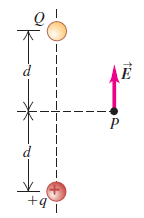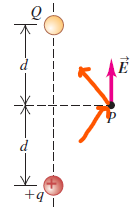×
Get Full Access to University Physics - 13 Edition - Chapter 21 - Problem 42e
Get Full Access to University Physics - 13 Edition - Chapter 21 - Problem 42e

×

# Two point charges Q and +q (where q is positive) produceISBN: 9780321675460 31

## Solution for problem 42E Chapter 21

University Physics | 13th Edition

• Textbook Solutions
• 2901 Step-by-step solutions solved by professors and subject experts
• Get 24/7 help from StudySoup virtual teaching assistantsUniversity Physics | 13th Edition

4 5 1 252 Reviews
10
3
Problem 42E

Two point charges Q and +q (where q is positive) produce the net electric field shown at point P in Fig. E21.36. The field points parallel to the line connecting the two charges. (a) What can you conclude about the sign and magnitude of Q? Explain your reasoning. (b) If the lower charge were negative instead, would it be possible for the field to have the direction shown in the figure? Explain your reasoning.

Step-by-Step Solution:

Q:Two point charges Q and +q (where q is positive) produce the net electric field shown at point P in Fig. E21.36. The field points parallel to the line connecting the two charges. (a) What can you conclude about the sign and magnitude of Q? Explain your reasoning. (b) If the lower charge were negative instead, would it be possible for the field to have the direction shown in the figure? Explain your reasoning.Step By Step Solution

Step 1 of 2:

(a)

Since the resultant electric field is parallel to the line connecting both the charges, the horizontal components will cancel, and the other component will point towards the charge Q and away from charge q . Hence the charge Q is negative.Step 2 of 2

##### ISBN: 9780321675460

Unlock Textbook Solution